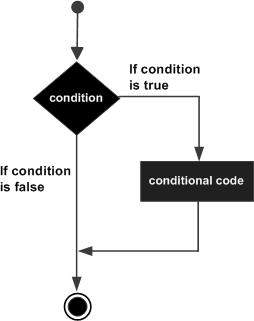# VB.Net - If ... Then语句

2018-12-16 13:45 更新

``````If condition Then
[Statement(s)]
End If
``````

``````If (a <= 20) Then
c= c+1
End If
``````

## 流程图：## 示例：

``````Module decisions
Sub Main()
'local variable definition
Dim a As Integer = 10

' check the boolean condition using if statement
If (a < 20) Then
' if condition is true then print the following
Console.WriteLine("a is less than 20")
End If
Console.WriteLine("value of a is : {0}", a)
End Sub
End Module
``````

``````a is less than 20
value of a is : 10
``````

App下载